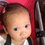# How to solve this?

So this problem was on our textbook and it seems that the infos given are not enough.

A sector of a circle has an arc of 16.76 cm and with chord 15.43 cm. Find the area of the sector.Note by Phak Mi Uph
5 years, 5 months ago

This discussion board is a place to discuss our Daily Challenges and the math and science related to those challenges. Explanations are more than just a solution — they should explain the steps and thinking strategies that you used to obtain the solution. Comments should further the discussion of math and science.

When posting on Brilliant:

• Use the emojis to react to an explanation, whether you're congratulating a job well done , or just really confused .
• Ask specific questions about the challenge or the steps in somebody's explanation. Well-posed questions can add a lot to the discussion, but posting "I don't understand!" doesn't help anyone.
• Try to contribute something new to the discussion, whether it is an extension, generalization or other idea related to the challenge.

MarkdownAppears as
*italics* or _italics_ italics
**bold** or __bold__ bold
- bulleted- list
• bulleted
• list
1. numbered2. list
1. numbered
2. list
Note: you must add a full line of space before and after lists for them to show up correctly
paragraph 1paragraph 2

paragraph 1

paragraph 2

[example link](https://brilliant.org)example link
> This is a quote
This is a quote
    # I indented these lines
# 4 spaces, and now they show
# up as a code block.

print "hello world"
# I indented these lines
# 4 spaces, and now they show
# up as a code block.

print "hello world"
MathAppears as
Remember to wrap math in $$ ... $$ or $ ... $ to ensure proper formatting.
2 \times 3 $2 \times 3$
2^{34} $2^{34}$
a_{i-1} $a_{i-1}$
\frac{2}{3} $\frac{2}{3}$
\sqrt{2} $\sqrt{2}$
\sum_{i=1}^3 $\sum_{i=1}^3$
\sin \theta $\sin \theta$
\boxed{123} $\boxed{123}$

Sort by:

You have $r\theta = 16.76$ and by cosine rule, $2r^2 - 2r^2 \cos\theta = 15.43^2$. So you have two equations and two variables, and you're instructed to find $\dfrac12r^2\theta$.

- 5 years, 5 months ago

im not good at solving variables involving theta

- 5 years, 5 months ago

Do you know numerical/approximation methods?

- 5 years, 5 months ago

don't know what is that.

- 5 years, 5 months ago

So you want to find $\dfrac12 r^2 \theta = \dfrac12 \cdot \dfrac{(r \theta)^2}r = \dfrac{16.76^2}{2\theta}$.

From the second equation, you have $2r^2(1-\cos\theta) =2r^2 \cdot 2\sin^2\left(\dfrac \theta2 \right) = 15.43^2 \Rightarrow 2r \sin \dfrac{\theta}2 = 15.43$.

$(r \theta) \div \left(2r \sin\dfrac\theta2 \right) = \dfrac{16.76}{15.43} \Rightarrow \dfrac{\theta /2}{\sin(\theta /2)} = \dfrac{1676}{1543}$

Let $y = \dfrac\theta2$, then you're left to solve for $\dfrac y{\sin y} = \dfrac{1676}{1543} \approx 1.08619$ for positive $y$ only as $\theta > 0$ as well.

Now for the numerical methods: Let $f(y) = \dfrac y{\sin y}$, and we want to find the best estimate of $y$ which gives $f(y) \approx 1.08619$.

Let's try for some value of $y$:

 y 0.5 0.6 0.7 0.8 0.9 f(y) 1.04291 1.06262 1.08659 1.11521 1.14895

Comparing all these values of $f(y)$ against the value $1.08619$, we can see that the closest value of $y$ that approximates to $f(y) \approx 1.08619$ is at $y = 0.7$. (Note that the more accurate reading is $y = 0.698497366525694\ldots$)

Thus we can make a rough estimate that $y \approx 0.7$ or $\dfrac \theta2 \approx 0.7 \Rightarrow \theta \approx 1.4$.

Our desired answer is approximately $\dfrac{16.76^2}{2\times1.4} = 100$.

Note that the actual value is $100.536\ldots$.

- 5 years, 5 months ago

thank you for the solution, my bad actually it is the area of the segment that I want to find and was needed (not sector). I used those two equations $2r^2-2r^2\cos\theta=15.43^2$ and $r\theta=16.76$ and I came up with $\large \frac{1-cos\theta}{\theta^2}=a$ where $\theta$ is in radian. (I forgot what the value of $a$ was) and used approximation. Result gives me $90>\theta>70$

- 5 years, 5 months ago

Your value of $\theta$ is measured in degrees, whereas my value of $\theta$ is measured in radians. $1.4 \text{ radians} \approx 80^\circ$.

- 5 years, 5 months ago

Yes. The $\theta$ in the latter part of my statement was in degrees. In radian that would be $\large \frac{\pi}{2}>\theta>\frac{7\pi}{18}$

- 5 years, 5 months ago

Yes, that's what I'm saying, the value you've found matches mine!

- 5 years, 5 months ago

Alternatively, you can use Newton's Method for estimating the value of y. Though there's something to note, newton's method would not work on certain functions.

- 5 years, 5 months ago

That's not the best way to teach someone about numerical methods. You should teach them about bisection method first. Newton-Raphson is way too complicated especially for someone who don't know calculus yet.

- 5 years, 5 months ago